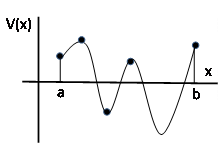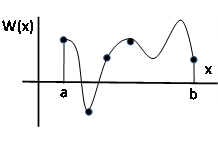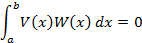Orthogonal

INTRODUCTION

In common usage the term “orthogonal” generally means unrelated or not relevant to the topic under discussion.  But the concept has ancient roots from the geometry used to survey land in our earliest civilizations.   More precisely, the etymology is a combination of the Greek roots “ortho” meaning straight and “gonia” meaning angle.   This was translated in Late Latin as “orthogonius” and comes to us to in its final form in French from the 1570s.   And its modern usage in mathematics is not only well defined but fundamental to many mathematical disciplines.

PERPENDICTULAR ANGLES

While orthogonal and perpendicular are effectively synonymous, orthogonal is the more general concept and perpendicular is restricted to discussions of angles.   A perpendicular angle is one that is 90 ° or π/2 radians.   Any triangle with a perpendicular angle such as a͞b͞c as shown below is called a “right” triangle.LINES

The equation of a line is given by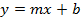where m is the slope and b is the y-axis intercept, i.e. the value of y when x = 0.

Note also that the equation of a line going through two points, such as (y1, x1) and (y2, x2), is thenSo for two lines as shown below, they are perpendicular when the product of their slops is (-1).For the above example, the equations of the two lines are given by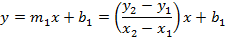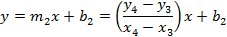and so the condition for the lines to be orthogonal is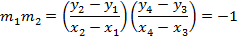QUADRATICS AND HIGHER ORDER POLYNOMIALS

If we have two quadratic functions, lacking rotational terms, as follows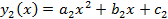Then the potential points of their intersections (xi  , yi) where from the well known equation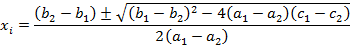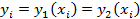And the slopes of the functions are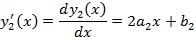And the quadratic functions are said to be orthogonal if the product of their slopes are -1 as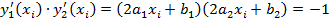These functions are illustrated below where the slopes are those of the straight line tangent to the functions at the point of intersection as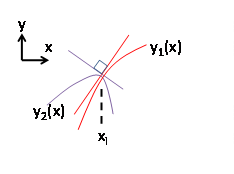For higher order polynomials, we have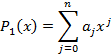VECTORS

Vectors have a magnitude and direction and are conventionally drawn as stretching from some origin (x, y) = (0,0) to some arbitrary point as shown below.The angle, α, between any two vectors is given by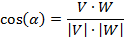and that they are perpendicular when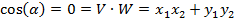Note that this is equivalent to the formula for two lines given above where the origin of the lines (x1, y1) and (x3, y3) would be set to zero.

FUNCTIONS

For more than two dimensions, perhaps n dimensions, the vectors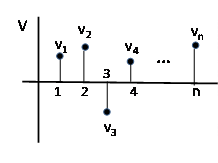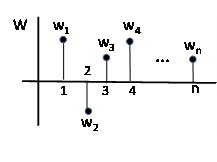Where the “inner” product is given by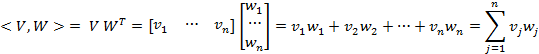And as n, the number of dimensions, becomes larger without limit the points begin to resemble a continuous curve